# Design of Retaining Wall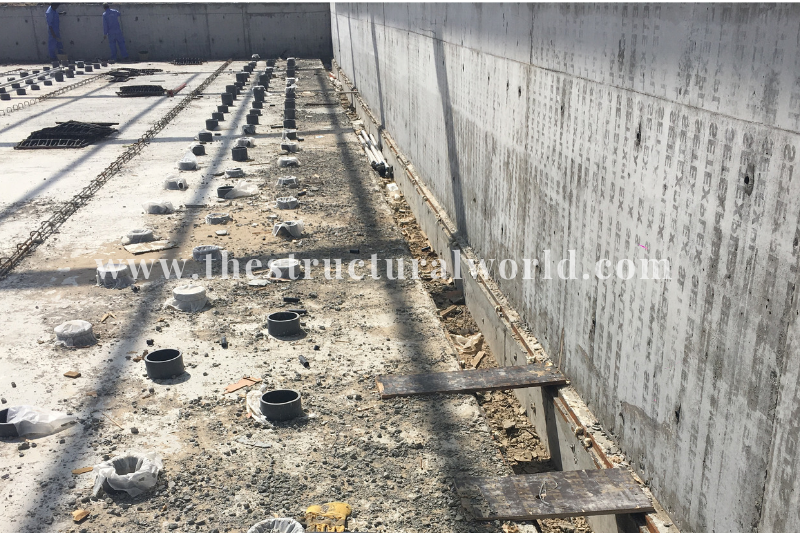Our previous article, Retaining Wall: A Design Approach discusses the principle and concept behind and when and where to consider a retaining wall in our design. We have learned the different checks against the mode of failures in the retaining wall should be considered in the design. To further understand the designed approach, here is a worked example of the design of retaining wall.

# Worked Example: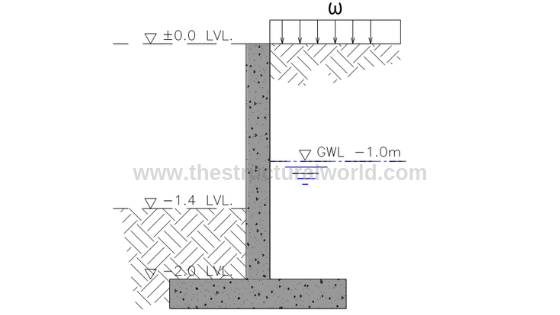Figure A.1-Retaining Wall Cross Section

Consider the cantilever retaining wall with the cross section shown in the above Figure A.1, which retain a 2m depth of soil having the groundwater table at -1.0m level.

## Design Parameters:

• Soil Bearing Capacity, qall : 100 kPa
• Coefficient of Soil Friction, ф: 30°
• Unit Weight of Soil, ɣs: 18 kN/m3
• Unit Weight of Water, ɣw: 10 kN/m3
• Unit Weight of Concrete, ɣc: 25 kN/m3
• Surcharge, ω: 12 kN/m2
• Ground Water Level: -1m from 0.00 level
• Height of Surcharge: 0.8m
• Height of Wall: 2.0m
• f’c: 32 Mpa
• fy: 460 Mpa
• concrete cover: 75mm

1. Analytical Geometry and Variables

Before we proceed with the design, it is important for the designer to know the geometric variable and parameters of the retaining wall. Refer to Figure A.2 below.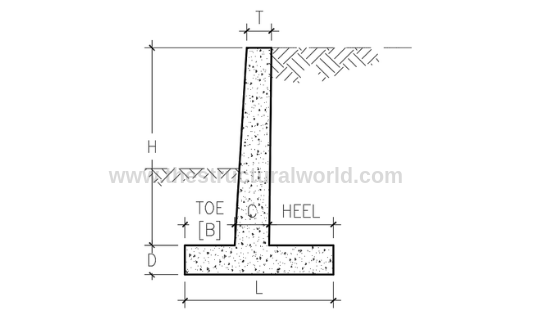Figure A.2-Retaining Wall Geometric Variables

where:

• H: Height of the retaining wall
• L: Width of the base
• D: Thickness of the base
• B: Width of toe
• C: Stem thickness at the bottom
• T: Stem thickness at the top

## 2. Approximate Proportions of a Cantilever Retaining Wall

The next thing to consider is the assumptions that we can make in terms of the geometry of the retaining wall that we are designing. Given the height, H of the retaining wall, we can assume or counter check our initial design considerations should at least according to the following geometric proportions:

• Base width: L= 0.5H to 2/3H
• Thickness of base: D= 0.10H
• Stem thickness at the bottom: C=0.10H
• Width of the toe: B= 0.25L to 0.33L
• Stem thickness at the top: t=250mm (minimum)

Based on the above approximate geometric proportions, let us assumed the following parameters to be used in our design:

• Base width:                 L= 1.5m
• Base thickness:          D= 0.25m
• Stem thickness:          C=t =0.25m
• Width of Toe:               B= 0.625m

## 3. Analytical Model

Sketches of the retaining wall forces should be considered to properly distinguish the different forces acting on our retaining wall as tackled in the previous article, Retaining Wall: A Design Approach. Based on our example in Figure A.1, we have the forces due to soil pressure, due to water and surcharge load to consider. Figure A.3 below is most likely our analytical model.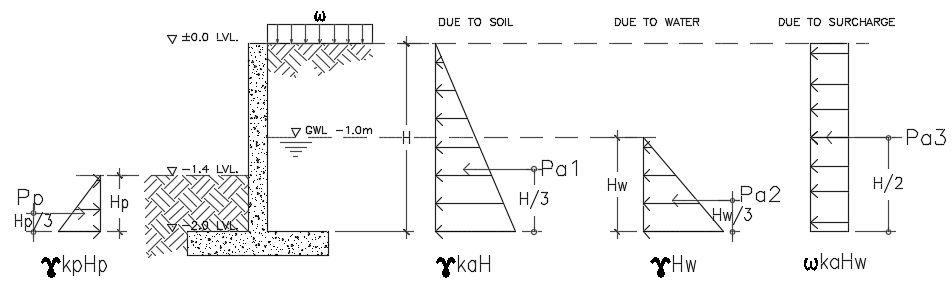READ ALSO:   Types of Foundations in Building Design

Figure A.3-Retaining Wall Forces Diagram

Considering the Figure A.3, we can derive the following equation for the active pressures, Pa and passive pressure Pp. Notice that the pressures acting on the wall are equivalent to the area of the pressure distribution diagram. Hence,

• Pa1=1/2 ɣkaH2              →eq. 1
• Pa2=1/2 ɣHw2                  →eq.2
• Pa3=ωkaH                    →eq.3

The passive pressure, Pp would be:

• Pp=1/2 ɣkpHp2               →eq.4

## Values of Coefficient of Pressure, ka and kp

According to Rankine and Coulomb Formula, the following are the equation in calculating the coefficient of pressure:

Ka= (1-sin ф)/(1+sin ф)

Ka= 0.33

Kp= (1+sin ф)/(1-sin ф)

Kp= 3

Substituting the values, we have the following results:

• Pa1=1/2 ɣkaH= 11.88kN
• Pa2=1/2 ɣHw2= 5kN
• Pa3=ωkaH= 3.17 kN
• Pp=1/2 ɣkpHp2= 9.72kN

## 3. Stability Check:

There are two checks to consider the stability of the retaining wall. One is the check for an overturning moment and the other one is the check for sliding. The weight of the retaining wall including the gravity loads within it plays a vital role in performing the stability check. Refer to Figure A.4 for the mass or weight calculations.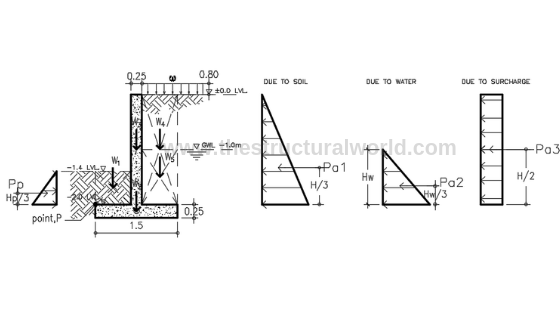Figure A.4-Retaining Wall Weight Components

The self-weight component of the retaining wall should be factored down or to be multiplied by weight reduction factor (0.9) to account for uncertainty because they are “stabilizing” in this context. Hence,

• Weight due to soil: W1= 18kN/m3 x 0.6m x 0.625m x 1.0m = 6.75kN
• Weight due to footing: W2= 0.9 x 25kN/m3 x 0.25m x 1.5m x 1.0m = 8.44kN
• Weight due to wall: W3= 0.9 x 25kN/m3 x 0.25m x 2.0m x 1.0m = 11.25kN
• Weight due to soil: W4= 18kN/m3 x 0.625m x 2.0m x 1.0m = 22.5kN
• Weight due to water: W5= 10kN/m3 x 0.625m x 1.0m x 1.0m = 6.25kN
• Weight due to surcharge: Ws= 18kN/m3 x 0.625m x 0.8m x 1.0m = 9kN
• Total Weight, WT = 64.19kN

### 3.1 Check for Overturning Moment:

To satisfy the Overturning Moment Stability, the following equation should follow:

where:

• RM: Righting Moment due to the weight of the retaining wall
• OM: Overturning Moment due to lateral earth pressure

With reference to the Figure A.4 diagram and taking moment at the point, P conservatively neglecting the effect of passive pressure hence:

• RM= W1 (0.313) + W2 (0.75) + W3(0.75) + W4(1.19) + W5(1.19) + Ws(1.19) = 61.80 kNm
• OM= Pa1 (0.67) +Pa2 (0.33) +Pa3 (0.4) = 10.88kNm

RM/OM = 5.68 > 2.0,                         hence SAFE in Overturning Moment!

### 3.2 Check for Sliding

To satisfy the stability against sliding, the following equation should govern:

where:

• RF: Resisting Force
• SF: Sliding Force
READ ALSO:   Pier and Spandrel Labeling for ETABS Shear Wall Design

The sliding check should be carried out with reference to the Figure A.4 diagram and considering the summation of vertical forces for resisting force and horizontal forces for sliding force conservatively neglecting the passive pressure, hence:

• RF= W1+W2+W3+W4+W5+ Ws = 64.19kN
• SF= Pa1+Pa2+Pa3 = 16.70kN

RF/SF = 3.84 > 1.5,      hence SAFE for Sliding!

## 4. Check the Wall Thickness for Shear

The nominal shear is equal to the lateral forces on the retaining wall, neglecting the effect of passive pressure which will give us:

• Nominal Shear, Vn = 20.05kN
• Ultimate Shear, Vu = 1.6Vn = 32.08kN

For the thickness of the wall to be safe in shear, the ultimate shear, Vu should less than the allowable shear, Vallow as recommended by the ACI 318 code.

Vc = 0.17√fc’bwd

where: ф=0.75

bw=1000mm

d= 250mm-75mm-6mm = 169mm

Vc = 0.17√fc’bwd = 162.52kN

Vallow= 121.89kN

Since Vu < Vallow, hence SAFE in Shear!

## 5. Design the Wall Stem for Flexure

• Nominal Moment, Mn = 10.88kNm
• Ultimate Moment, Mu = 1.6Mn = 17.40kNm

Mu =φ fc’ bd2ω (1- 0.59 ω)

17.40×106 = 0.90 x 32 x 1000 x 1692 ω (1-0.59 ω)

ω = 0.0216

ρ = ω fc’/fy= 0.00150

As= ρbd = 0.00150x1000x169 = 254mm2

Asmin= ρminbt = 0.002 x 1000 x 250 = 500mm2

Required Vertical Bar: Try T10-200; As act= 392mm2 x 2 sides = 785.4mm2

Required Horizontal Bar: Try T10-250; As act= 314mm2 x 2 sides= 628.32mm2

Hence: use T10-200 for vertical bar and T10-250 for horizontal bar.

## 6. Check for Bearing Pressure under Footing

The foundation bearing capacity usually governs the design of the wall. The soil, particularly under the toe of the foundation, is working very hard to resist the vertical bearing loads, sliding shear, and to provide passive resistance to sliding. The bearing capacity of the soil should be calculated taking into account the effect of simultaneous horizontal loads applied to the foundation from the soil pressure.

For the footing to be safe in soil pressure, the maximum soil pressure under working load shall be less than the allowable soil bearing capacity. The maximum soil bearing pressure under the footing considering 1m strip is:

where:

• P= 64.19kN
• A= (1×1.5) m2
• M=10.88 kNm
• b= 1m
• d=1.5m

Substituting the values above will give us:

qmax= 71.81kPa < qall=100 kPa, hence, oK!

Solving for Ultimate bearing pressure:

where:

• P= 1.6x 6.75 + 1.4×8.44 +1.4×11.25 +1.6x 22.5 +1.6×6.25 +1.6×9= 98.76kN
• A= (1×1.5) m2
• M=17.40kNm
• b= 1m
• d=1.5m

Substituting the values above will give us:

• qumax= 112.24kN
• qumin= 19.44kN

## 7. Check the Required Length of the Base

If qumin is in tension check the required length otherwise ignore if it is in compression. Since our qumin is tension (+), the value of L must be computed as follows: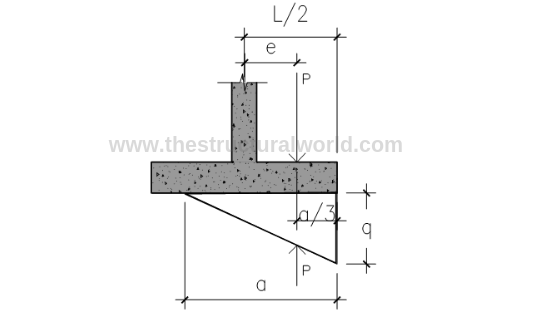Figure A.5-Pressure Diagram under Tension

From Figure A.5:

Solve for Eccentricity:

e=M/P = 0.176

where:

• a=length of pressure
• qe= qumax
• b=1 meter strip

• a= 1.75m

L= 2(e+a/3) = 1.52 say 1.6m

## 8. Check the Adequacy of Footing Thickness for Wide Beam Shear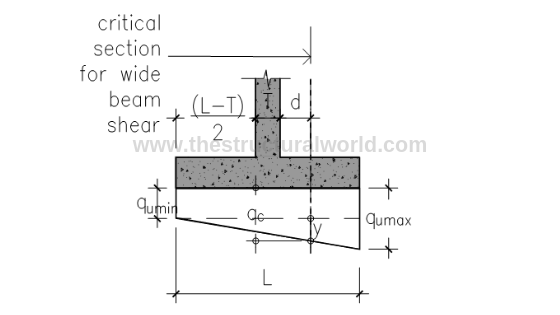Fig A.6-Pressure Diagram under Compression

### 8.1 When qumin is in Compression

Solving for y by similar triangle: referring to Figure A.6 above

y/1.044      = (112.24-19.44)/1.5;        y = 64.59 kPa

qc= 19.44 + 64.59 = 84.03 kPa

• L’= (1.5m-1.044m) = 0.456m
• B= 1m strip
• qumax=112.24kPa

Vu= 44.75kN

### 8.2 When qumin is in Tension

qc=y

Solving for y by similar triangle: (referring to Figure A.6 above, L=a=1.75)

y/1.244= 112.24/1.75;         y = 79.79 kPa

qc=79.79kPa

Vu= 1/2 (qc + qumax) L’b

• L’= (1.6m-1.244m) = 0.356m
• B= 1m strip
• qumax=112.24 kPa

Vu=34.18kN

Hence, use:  Vu=44.75kN

Vallow= фVc

Vc = 0.17√fc’bwd

where:

• ф=0.75
• bw=1000mm
• d= 250mm-75mm-6mm = 169mm

Vc = 0.17√fc’bwd = 162.52kN

Vallow= 121.89kN

Since Vu < Vallow, hence SAFE in Shear!

## 9. Check the Wall Thickness for Flexure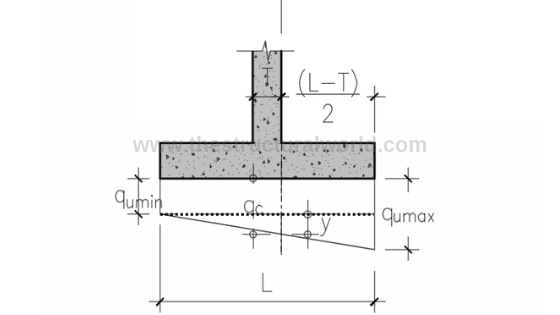Figure A.7-Pressure Diagram for Flexure Check

### 9.1 When qumin is in Compression

Solving for y by similar triangle:(referring to Figure A.7 above)

y/0.875      = (112.24-19.44)/1.5;        y = 54.13 kPa

qc= 19.44 + 54.13 = 73.57 kPa

Mu= (73.57×0.625) x (0.625/2) + (38.67×0.625) (1/2) x (2/3) (0.625) → (area of trapezoid x lever arm)

Mu=19.40 kNm

### 9.2 When qumin is in Tension

qc= qumin + y

Solving for y by similar triangle: (referring to Figure A.7 above. L=a=1.75)

y/1.075    = 112.24/1.75;         y = 68.95 kPa

qc=19.44 + 68.95= 88.39 kPa

Mu = (88.39×0.75) x (0.75/2) + (23.85×0.75) (1/2) x (2/3) (0.75) → (area of trapezoid x lever arm)

Mu= 19.40kNm

Hence, use Mu=29.33kNm

Mu =φ fc’ bd2ω (1- 0.59 ω)

29.33×106 = 0.90 x 32 x 1000 x 1692 ω (1-0.59 ω)

ω = 0.0364

ρ = ω fc/fy= 0.002532

As= ρbd = 0.002532x1000x169 = 428mm2

Asmin= ρminbt = 0.002 x 1000 x 250 = 500mm2

Required Vertical Bar: Try T10-200; As act= 392mm2 x 2 sides = 785.4mm2

Required Horizontal Bar: Try T10-250; As act= 392mm2 x 2 sides= 628.32mm2

## 10. Reinforcement Details of Retaining Wall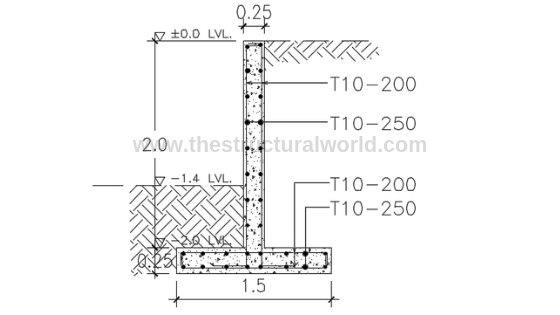The presented calculations above are actually too tiring to perform manually especially if you are doing a trial and error design. Thanks to structural design soft wares and spreadsheets, available nowadays, our design life will be easier.

Our team developed a user-friendly spreadsheet for the design of cantilever retaining wall based on the above calculation. Grab your copy here!

13,312 total views, 12 views todayCopyright secured by Digiprove © 2019 The Structural World

1.Mohmand Andar

i need for your help minimum depth for stone masonry wall refer to ACI code is how much?
kindly send me with Ref of ACI code

1.thestructuralworld (Post author)

You can use the parameters written in section 2 of this article and confirm whether the thickness is adequate using a shear check.

2.Ross

This is a helpful guide to Retaining Wall design. However, I have noticed errors in Section 3’s loadings. For example, Pp is not substituted correctly. I have not checked further than this.

It would be worth double-checking the spreadsheet you are selling as this is a dangerous error that would show a retaining wall having a higher capacity than it actually does.

1.thestructuralworld (Post author)

Thanks for pointing out. We have checked and found out that that is merely a typo error and it has been updated accordingly. We have also double-checked the attached spreadsheet and it is not affecting the results as we conservatively neglect the effect of passive pressure in the calculation.

3.Intisar

Helpful guide. Which section in ACI360 the calculations are referred to?

4.Edgar

Hello

Can you explain why the height of the surcharge is only 0.8m and why is it not reflected on the Analytical model ?

Its very detailed example , thanks for that.

1.Edgar

Also ,would you be able to explain how is the d in critical shear calculated ? number 1.044 is used for similar triangles,however I struggle to find exact theory how you arrived to this number as I get different.

1.thestructuralworld (Post author)

Substitute the values from (L-T)/2 + T + d, where L=1.5, T=0.25 and d=.169. That will give us 1.044.

2.thestructuralworld (Post author)

In this example, a 0.80m surcharge height is a given parameter. In the analytical model, the surcharge, ω is mentioned and the height corresponds to that is 0.80 m.

5.Rosina

Very helpful information.. thanks for sharing

1.thestructuralworld (Post author)

Thanks Rosina!

All original content on these pages is fingerprinted and certified by Digiprove